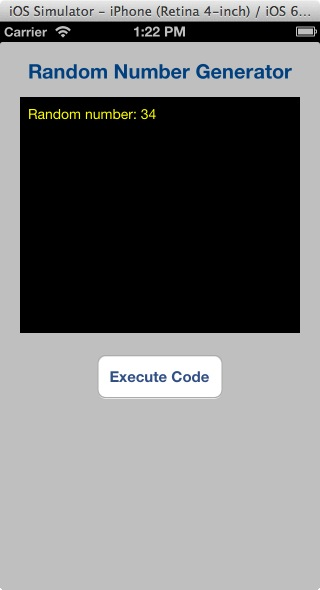If you are a new visitor to our site, please read the Getting Started guide.

In this workshop, you will learn how to do the following:
☻ Generate and display random numbers
☻ Generate and display random numbers in a specified range

### Before You Begin

I’ve created an Xcode project you can use to practice code provide in this workshop, so go ahead and download and unzip it now. Drop the RandomNumberGenerator folder in the iOS Apps folder, then launch it in Xcode.

### Generate and display random numbers

Random numbers are useful in many programming situations. In particular, games and quizzes need random numbers to make the applications interesting. You do not want to be presented with a deck of cards dealt in the same sequence in every game. Similarly, to have an effective quiz, you might not want to be asked questions in the same order every time you attempt the quiz.

The C library provides the function, rand(), for generating random numbers. The number returned by the function is an int. Here is an example of how to use the rand() function:
`randomNumber = rand();`

After this statement executes, the variable randomNumber will contain a random number. The numbers generated by this function aren’t truly random; they are pseudo random; that is, the function uses an algorithm that produces the same sequence of numbers each time the application runs on the same device. For example, suppose the following statements are executed.

```- (IBAction)executeCodeButtonClicked {
secondRandomNum = rand(),
thirdRandomNum = rand();

}```

These three numbers are displayed in the textView control and appear to be random, but each time you run the application, the same three values will be generated.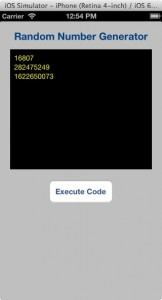In order to randomize the results of rand(), the srand() function must be used. The srand() function accepts an int argument, which acts as a seed value for the algorithm. By specifying different seed values, rand() will generate different sequences of random numbers. Here’s how you’d use the srand() and rand() function in the executeCodeButtonClicked method and output the method will generate in the textView control.

##### Listing 1
```- (IBAction)executeCodeButtonClicked {
// Seed the random number generator
int seed = 47 ;
srand(seed);

// Format and display a random number
self.textView.text = [NSString stringWithFormat:@"Random number %i",rand()];
}
```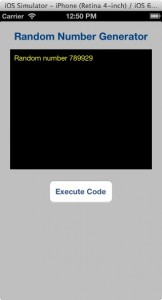A common way to more truly randomized generated numbers is to use the time() function. It returns the number of seconds that have elapsed since midnight, January 1, 1970. Because the time will always be different every time the application is launched, the sequence of generated numbers will always be different. You pass NULL as an argument to the time() function. Code Listing 2 demonstrate how to use the srand() and time() function to generate three different random numbers each time the application is launched. Output shows a sample run of the application.

##### Listing 2
Output
```- (IBAction)executeCodeButtonClicked {
// Get the device's time
int seed = time(NULL);

// Seed the random number generator
srand(seed);

// Create and display three random numbers
int firstRandomeNum = rand(), secondRandomNum = rand(), thirdRandomNum = rand();
self.textView.text = [NSString stringWithFormat:@"%i\n%i\n%i",
}
```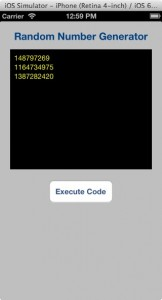### Generate and display random numbers in a specified range

Code in Listing 2 generate and display random numbers in no particular range. These numbers may be to large for practical use. For example, you might only need random numbers in the range 0 through 9 to use as a subscript to randomly choose an item stored in an array. There are many ways to generate numbers in a specified range. One of the simplest method is to use the modulus [%] operator and a value that is one more than the highest number you want. For example, if you wish to limit the range of a random number, us the following formula.
`result = 1 + (rand() % maxRange);`

The maxRange value is the upper limit of the range. For example, if you wish to generate a random number in the range of 1 through 100, use the following statements.``` result = 1 + (rand() % maxRange); ```

This is how the statement works:

Look at the following expression.
`rand() % 100`

Assuming rand( ) returns 37894, the value of the expression above is 94. That is because 37894 divided by 100 is 378 with a remainder of 94. (The modulus operator returns the remainder.) But, what if rand() returns a number that is evenly divisible by 100, such as 500? The expression above will return 0. If we want a number in the range 1 – 100, we must add 1 to the result. That is why we use the expression 1 + rand() % 100.

Listing 3 demonstrates what was said above and the image below shows result generated when you click the Execute Code button.

##### Listing 3
Output
```- (IBAction)executeCodeButtonClicked {
const int maxRange = 100;

// Generate a random number
srand(time(NULL));

// Place remainder of the expression in the result variable
int result = 1 + rand() % maxRange;

// Format and display the variable's value in the textView control
self.textView.text = [NSString stringWithFormat:@"Random number: %i", result];
}
```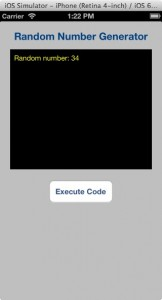Categories:

Tags:

#### No responses yet

###### UIDocument Demystified: A Step-by-step Guide on Local and iCloud Document Storage